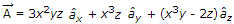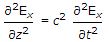# Electronics and Communication Engineering - Electromagnetic Field Theory

1.
For a distortionless line, the parameters are related as
R/G = L/C
R/L = 1
R/G = C/L
RG = LC
Explanation:
No answer description is available. Let's discuss.

2.
A fieldcan be
harmonic
divergence less
solenoidal
rotational
Explanation:
No answer description is available. Let's discuss.

3.
Phase velocity of waves propagating in a hollow metal waveguide is
greater than the group velocity
less than the velocity of light in free space
equal to the velocity of light in free space
equal to group velocity
Explanation:
No answer description is available. Let's discuss.

4.
If a plane electromagnetic wave satisfies the equation, the wave propagates in the (at an angle of 45° between the x and z direction)
x-direction
z-direction
y direction
xy plane